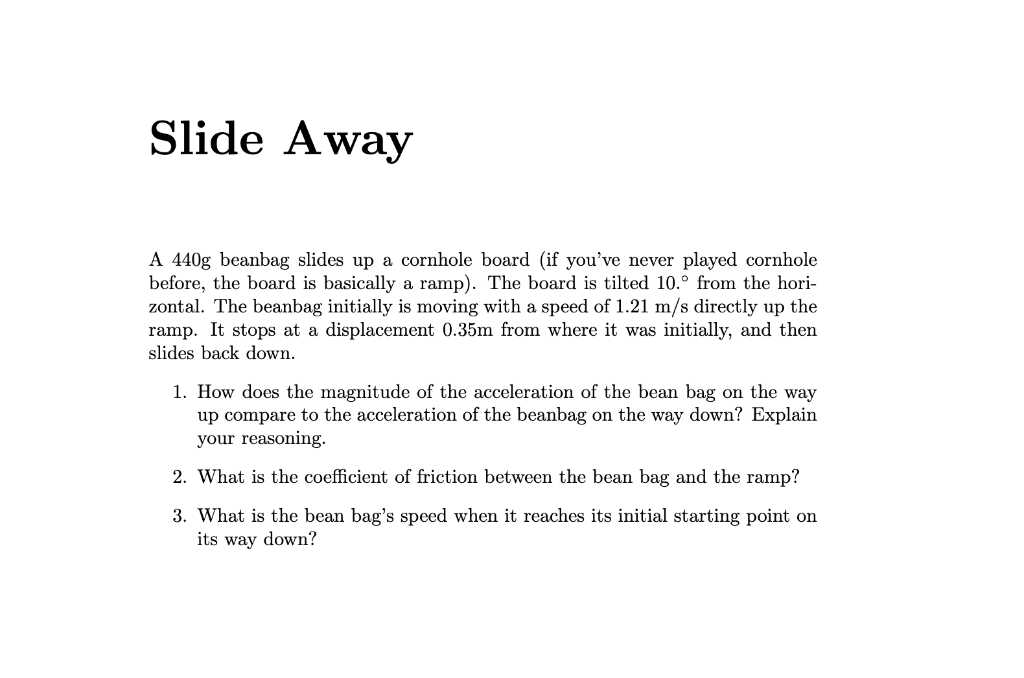# Question Slide Away A $$440 \mathrm{~g}$$ beanbag slides up a cornhole board (if you've never played cornhole before, the board is basically a ramp). The board is tilted $$10 .^{\circ}$$ from the horizontal. The beanbag initially is moving with a speed of $$1.21 \mathrm{~m} / \mathrm{s}$$ directly up the ramp. It stops at a displacement $$0.35 \mathrm{~m}$$ from where it was initially, and then slides back down. 1. How does the magnitude of the acceleration of the bean bag on the way up compare to the acceleration of the beanbag on the way down? Explain your reasoning. 2. What is the coefficient of friction between the bean bag and the ramp? 3. What is the bean bag's speed when it reaches its initial starting point on its way down?Transcribed Image Text: Slide Away A $$440 \mathrm{~g}$$ beanbag slides up a cornhole board (if you've never played cornhole before, the board is basically a ramp). The board is tilted $$10 .^{\circ}$$ from the horizontal. The beanbag initially is moving with a speed of $$1.21 \mathrm{~m} / \mathrm{s}$$ directly up the ramp. It stops at a displacement $$0.35 \mathrm{~m}$$ from where it was initially, and then slides back down. 1. How does the magnitude of the acceleration of the bean bag on the way up compare to the acceleration of the beanbag on the way down? Explain your reasoning. 2. What is the coefficient of friction between the bean bag and the ramp? 3. What is the bean bag's speed when it reaches its initial starting point on its way down?
Transcribed Image Text: Slide Away A $$440 \mathrm{~g}$$ beanbag slides up a cornhole board (if you've never played cornhole before, the board is basically a ramp). The board is tilted $$10 .^{\circ}$$ from the horizontal. The beanbag initially is moving with a speed of $$1.21 \mathrm{~m} / \mathrm{s}$$ directly up the ramp. It stops at a displacement $$0.35 \mathrm{~m}$$ from where it was initially, and then slides back down. 1. How does the magnitude of the acceleration of the bean bag on the way up compare to the acceleration of the beanbag on the way down? Explain your reasoning. 2. What is the coefficient of friction between the bean bag and the ramp? 3. What is the bean bag's speed when it reaches its initial starting point on its way down?